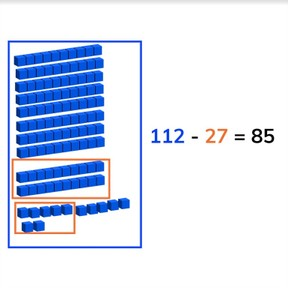Subtraction through 100 regrouping tens

# Subtraction through 100 regrouping tens

No account needed.8,000 schools use Gynzy92,000 teachers use Gynzy1,600,000 students use Gynzy

## General

Students learn to subtract numbers to 100 regrouping tens.

2.NBT.B.5

## Relevance

It is important to be able to subtract numbers through 100, so you can determine how many you have left.

## Introduction

Start by practicing with practicing numbers on the number line to 100 by giving each student a sheet of paper and asking them to write a number between 0 and 100. They must then come to the front of the class with their numbers and form a number line in silence. Where do they belong? Are there any doubles? Next practice a subtraction problem on the number line to 100.

## Development

Start by discussing the importance of subtracting through one hundred. Next the learning goal is given in three ways, visually, in the abstract, and as story problems. You can navigate beween these three by using the blue menu on the bottom right. You can select the method which best meets the learning needs of your class. The lesson starts with the visual method. Using the blocks on the interactive whiteboard explain how to solve a subtraction problem. Have students practice subtraction problems with visual support. Then discuss the abstract subtraction problems. Tell students that they can solve these problems by counting back or by counting back in jumps. Using a number line can be very helpful here. Have students practice abstract problems individually or in pairs. Finally discuss the steps of solving a story problem, namely that they must determine what kind of math problem it is, which numbers are important for the problem, to say or write the problem and then to solve the problem. Solve one story problem as a class and then have students solve a story problem individually or in pairs.

Check that students are able to subtract numbers through 100 without regrouping tens by asking the following questions:
- Why is it useful to be able to subtract through 100?
- How do you solve the subtraction problem 112-32? Which steps do you take?

## Guided practice

Students first stat with visually supported subtraction problems, then abstract and then are given story problems to practice.

## Closing

Discuss the importance of subtracting through 100 with the class and ask students to solve three subtraction problems, one visual, one abstract, and one story problem. To close the lesson divide the classroom in half. Half of the students must write a number between 100 and 150 and the other half must write a nubmer between 50 and 100. They must then form pairs (with an opposite half member) and create a subtraction problem which they then must solve. Repeat this swapping the numbers the students have to write. Ask some pairs to tell their subtraction problems and how they found the difference.

## Teaching tips

Students who have difficulty with this learning goal can make use of manipulatives or use the number line to show the subtraction problem.

## Instruction materials

Optional: Blocks or other manipulatives.

### The online teaching platform for interactive whiteboards and displays in schools

• Save time building lessons

• Manage the classroom more efficiently

• Increase student engagement